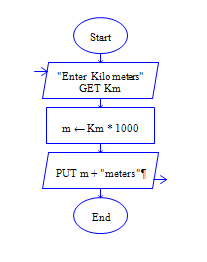# Java Program to Meters to Kilo Meters

Get meter m from user and find kilometer km

Sample Input 1:

56000

Sample Output 1:

56

#### Flow Chart Design#### Program or Solution

``` import java.util.*; class Convertor { public static void main(String args[]) { float km,metres; Scanner sc=new Scanner(System.in); System.out.println("Enter The Meters:"); metres=sc.nextInt(); km=metres/1000; System.out.println("The Output 1 Is:\n"+km); } } ```

#### Program Explanation

Get meter mts as input (using scanner class)

Calculate kilometer km by dividing meter mts by 1000.

(km=mts/1000, 1000 meter is equal to 1 kilometer)